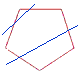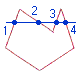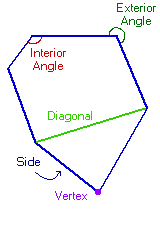Home    |    Teacher    |    Parents    |    Glossary    |    About UsEmail this page to a friendResources· Cool Tools · Formulas & Tables · References · Test Preparation · Study Tips · Wonders of MathSearchPolygon Properties (Math | Geometry | Polygon Properties)

What is a Polygon?
A closed plane figure made up of several line segments that are joined together. The sides do not cross each other. Exactly two sides meet at every vertex.

Types of Polygons
Regular - all angles are equal and all sides are the same length. Regular polygons are both equiangular and equilateral.
Equiangular - all angles are equal.
Equilateral - all sides are the same length.Convex - a straight line drawn through a convex polygon crosses at most two sides. Every interior angle is less than 180°.Concave - you can draw at least one straight line through a concave polygon that crosses more than two sides. At least one interior angle is more than 180°.

Polygon Formulas
(N = # of sides and S = length from center to a corner)

Area of a regular polygon = (1/2) N sin(360°/N) S2

Sum of the interior angles of a polygon = (N - 2) x 180°

The number of diagonals in a polygon = 1/2 N(N-3)
The number of triangles (when you draw all the diagonals from one vertex) in a polygon = (N - 2)

Polygon PartsSide - one of the line segments that make up the polygon. Vertex - point where two sides meet. Two or more of these points are called vertices. Diagonal - a line connecting two vertices that isn't a side. Interior Angle - Angle formed by two adjacent sides inside the polygon. Exterior Angle - Angle formed by two adjacent sides outside the polygon.

Special Polygons
Special Quadrilaterals - square, rhombus, parallelogram, rectangle, and the trapezoid.

Special Triangles - right, equilateral, isosceles, scalene, acute, obtuse.

Polygon Names
Generally accepted names

 Sides Name n N-gon 3 Triangle 4 Quadrilateral 5 Pentagon 6 Hexagon 7 Heptagon 8 Octagon 10 Decagon 12 Dodecagon

Names for other polygons have been proposed.

 Sides Name 9 Nonagon, Enneagon 11 Undecagon, Hendecagon 13 Tridecagon, Triskaidecagon 14 Tetradecagon, Tetrakaidecagon 15 Pentadecagon, Pentakaidecagon 16 Hexadecagon, Hexakaidecagon 17 Heptadecagon, Heptakaidecagon 18 Octadecagon, Octakaidecagon 19 Enneadecagon, Enneakaidecagon 20 Icosagon 30 Triacontagon 40 Tetracontagon 50 Pentacontagon 60 Hexacontagon 70 Heptacontagon 80 Octacontagon 90 Enneacontagon 100 Hectogon, Hecatontagon 1,000 Chiliagon 10,000 Myriagon

To construct a name, combine the prefix+suffix

 Sides Prefix 20 Icosikai... 30 Triacontakai... 40 Tetracontakai... 50 Pentacontakai... 60 Hexacontakai... 70 Heptacontakai... 80 Octacontakai... 90 Enneacontakai...
+
 Sides Suffix +1 ...henagon +2 ...digon +3 ...trigon +4 ...tetragon +5 ...pentagon +6 ...hexagon +7 ...heptagon +8 ...octagon +9 ...enneagon

Examples:
46 sided polygon - Tetracontakaihexagon

28 sided polygon - Icosikaioctagon

However, many people use the form n-gon, as in 46-gon, or 28-gon instead of these names.